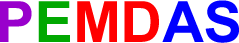Which is it?

Both answers can't be right or we'd always be arguing about the answers to math problems.  The nice thing about math is that there's always just ONE answer!

So, a long time ago, math geeks decided to make a set of rules for what to do first in a math problem.

These rules are called "the order of operations."

I'll tell you what the rules are in the next lessons...  For now, remember this word:Go to the next lesson to find out if the answer to our problem is 7 or 9!  And go all the way through to find out what all the letters of PEMDAS stand for!